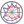Course title
Calculus with Differential Equations
 TAKEUCHI ShingoCourse description
You will learn what a differential equation is and how to recognize some of the basic different types. You will learn how to apply some common techniques used to obtain general solutions of differential equations and how to fit initial or boundary conditions to obtain a unique solution. You will appreciate how differential equations arise in applications and you will gain some experience in applying your knowledge to model a number of engineering problems using differential equations.
Purpose of class
The purpose of this class is to learn how to recognize some of the basic different types of differential equations, to learn how to apply some common techniques used to obtain solutions of differential equations and to appreciate how differential equations arise in applications. This class also includes a review on the content learned in the class of differential equations at the time of first grade.
Goals and objectives
1. You can describe how to recognize some of the basic different types of differential equations
2. You can describe how to apply some common techniques used to obtain solutions of differential equations
3. You can describe how differential equations arise in applications
Language
English
Class schedule

Class schedule HW assignments (Including preparation and review of the class.) Amount of Time Required
1. Orientation, Case study of modelling Exercises for the models 190minutes
2. General solution Review general solutions 190minutes
3. Method of separation of variables Applying the method of separation of variables 190minutes
4. Inverse function Review inverse functions 190minutes
5. Exact equation Solving exact equations 190minutes
6. Finding the Integrating factor Solving equations via integrating factors 190minutes
7. Bernoulli differential equation Review Bernoulli differential equations 190minutes
8. Attention on Mid-term assignment Review Sessions 1-7 190minutes
9. 2nd order constant coefficient equations Finding complementary functions 190minutes
10. 2nd order constant coefficient equations: exercise Finding complementary functions 190minutes
11. Particular integral Finding particular integrals 190minutes
12. General solution of inhomogeneous case Finding general solutions 190minutes
13. Applications Exercises for the models 190minutes
14. Attention on Final assignment Review Sessions 9-13 190minutes
Total. - - 2660minutes
Relationship between 'Goals and Objectives' and 'Course Outcomes'

Mid-term assignment Final assignment Total.
1. 20% 20% 40%
2. 20% 20% 40%
3. 10% 10% 20%
Total. 50% 50% -
Evaluation method and criteria
Mid-term assignment and Final assignment. As a criterion, if you determine that you understand 60% of the content covered in the class, your final score will be 60 points.
Textbooks and reference materials
No textbook
Prerequisites
Basic knowledge of calculus is required
Office hours and How to contact professors for questions
• Lunchtime on every Tuesday.
Regionally-oriented
Non-regionally-oriented course
Development of social and professional independence
• Course that cultivates an ability for utilizing knowledge
Active-learning course
More than one class is interactive
Course by professor with work experience
Work experience Work experience and relevance to the course content if applicable
N/A 該当しない
Education related SDGs:the Sustainable Development Goals• 4.QUALITY EDUCATION